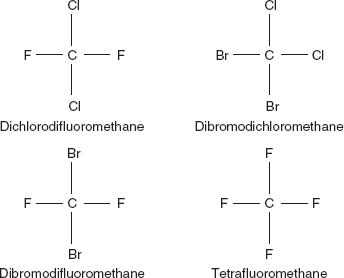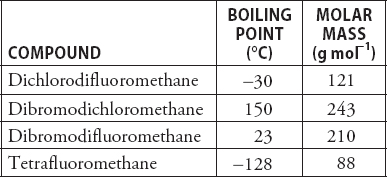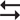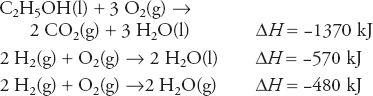# AP Chemistry Practice Test 46

### Test Information11 questions16 minutes

1. A solution of a weak base is titrated with a solution of a standard strong acid. The progress of the titration is followed with a pH meter. Which of the following observations would occur?

2. A student mixes 100.0 mL of a 0.10 M hydrofluoric acid, HF, solution with 50.0 mL of a 0.10 M potassium hydroxide, KOH, solution. The Ka for hydrofluoric acid is 6.8 × 10-4. Which of the diagrams below best represents the species, other than H2O, in the solution after the acid reacts with the base?

3. Which of the following best represents the result for the reaction of 50.0 mL of a 0.20 M barium hydroxide, Ba(OH)2, solution with 50.0 mL of a 0.10 M sulfuric acid, H2SO4, solution to form a precipitate of BaSO4?

4. The container in the above diagram had an initial volume of 10.0 L. The container was heated until the temperature was double the original temperature. After heating, the pressure was measured and the piston moved down until the pressure was four times the original value. What was the final volume of the container?

5.The diagram above shows the structure of molecules of CCl4 and CBr4 and the above table gives the boiling points and molar masses of the compounds. Which of the compounds is nonpolar?

6. 2 SO2(g) + O2(g)2 SO3(g)

The reaction above is allowed to continue until equilibrium is established. After equilibrium is established a catalyst is added to the system. How does the rate of the forward reaction compare to the rate of the reverse reaction after the addition of the catalyst?

7. A reaction has the following suggested mechanism:

1. NH3(aq) + OCl-(aq) → NH2Cl(aq) + OH-(aq)

2. NH3(aq) + NH2Cl(aq) → Cl-(aq) + N2H5+(aq)

3. OH-(aq) + N2H5+(aq) → N2H4(aq) + H2O(l)

Referring to the above mechanism, which of the following would support the suggested mechanism?

8. Determine the final temperature, in °C, of a sample of helium gas. The sample initially occupied a volume of 5.00 L at 127°C and 875 mm Hg. The sample was heated, at constant pressure, until it occupied a volume of 10.00 L.

9. A solution contains 2.00 mole of acetic acid, CH3COOH, and 1.00 mole of calcium acetate, Ca(CH3COO)2. The solution is able to resist the addition of a small amount of strong acid or strong base with only minor changes in the pH of the solution. Larger quantities of strong acid or strong base can cause a significant change in pH. How many moles of nitric acid, HNO3, may be added before the pH begins to change significantly?

`Questions 10-11 refer to the following information.Use the following information in the following thermochemical equations to answer questions.`

10. Determine ΔH for the combustion of ethanol, C2H5OH, if H2O(g) formed in the above reaction instead of H2O(l).

11. What is the energy change when 72.0 g of water vapor decomposes to the elements at constant pressure? The molar mass of H2O is 18.0 g mol-1.